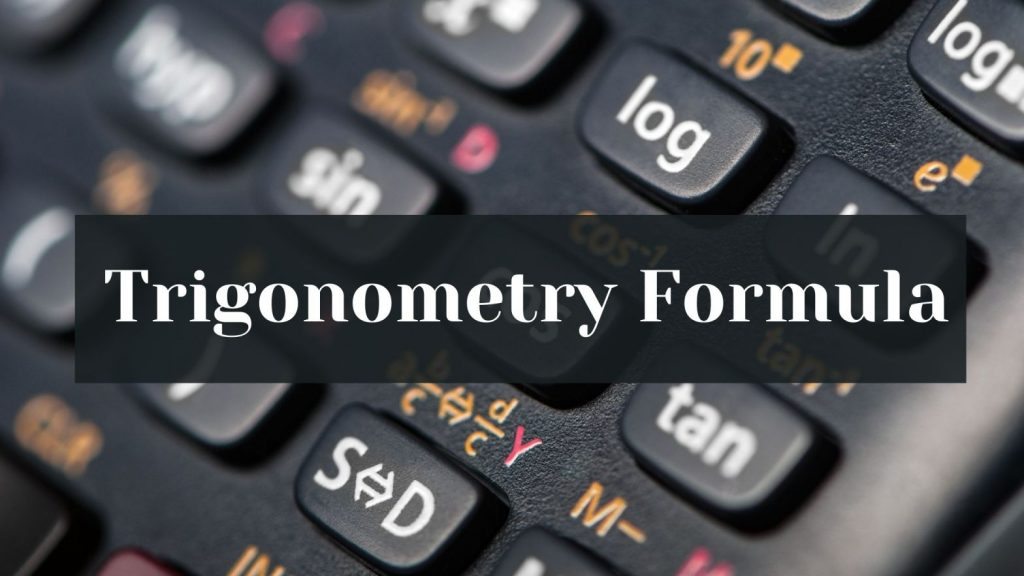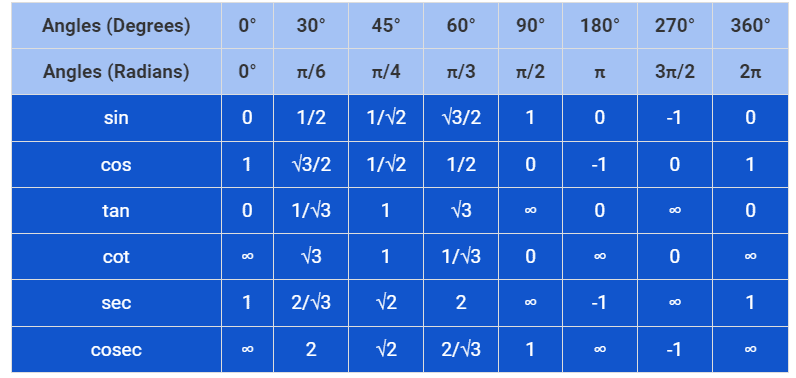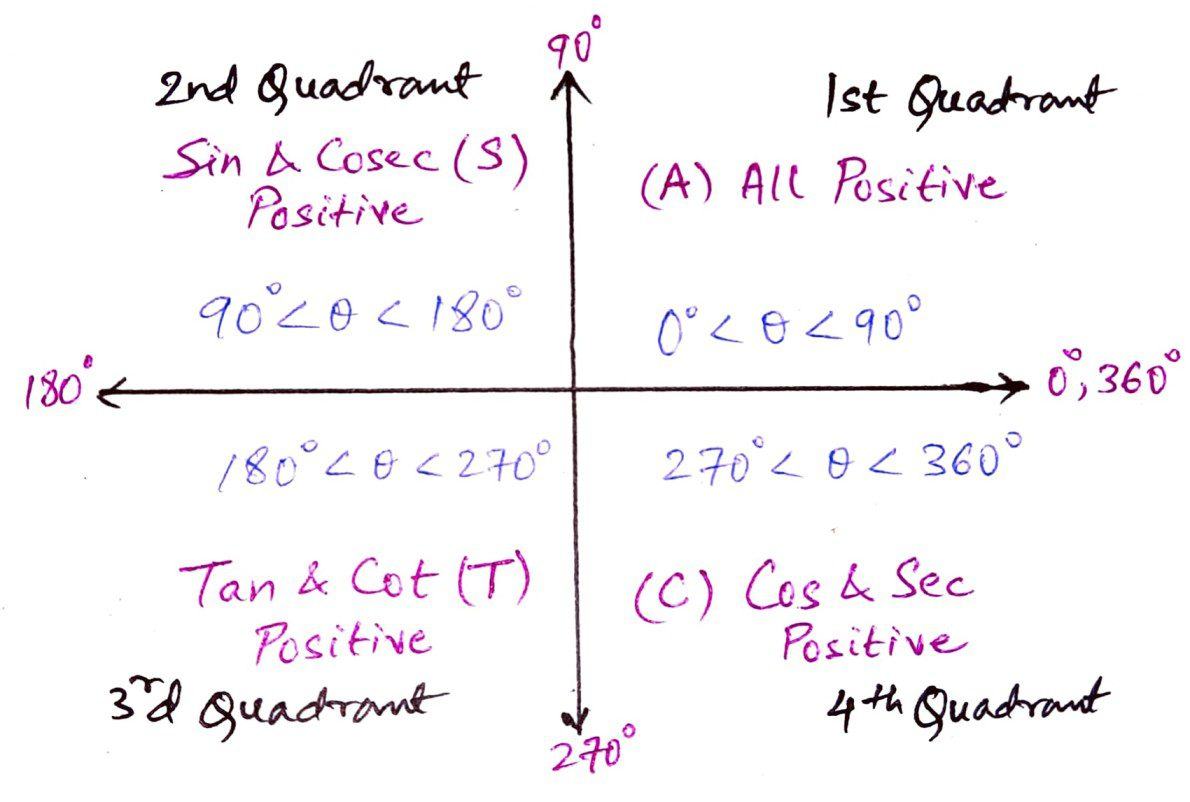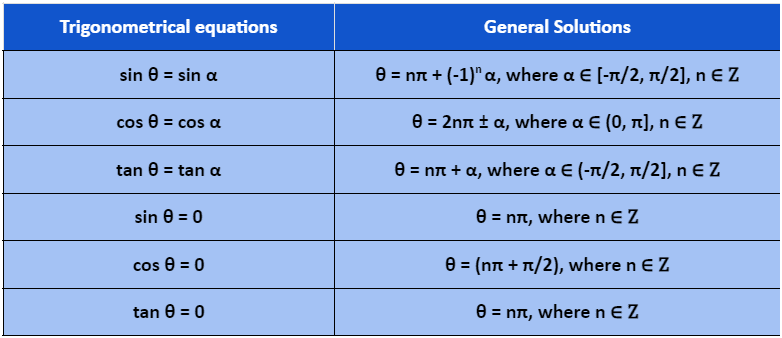Maths
Study Material

# Trigonometry Formula(s) and Identities | Complete List |

The word ‘trigono’ means ‘triangle’ and ‘metry’ means ‘measure’. Therefore, the word ‘Trigonometry’ means ‘The study of the measurement of triangles’.

6 minutes long
Posted by Kunduz Tutor, 13/10/2021Hesap Oluştur

Got stuck on homework? Get your step-by-step solutions from real tutors in minutes! 24/7. Unlimited.

Trigonometry formula(s) is a set of various formulas and trigonometric identities that can be used to solve the problems on trigonometric equations, sides and angles of a right-angled triangle. In fact, numerous problems can be solved with the help of trigonometry formula(s) and trigonometric identities in Trigonometry.

Memorizing the concepts of trigonometry, especially trigonometry formula and trigonometric identities in mathematics, will be fruitful for the learners of classes 10, 11 and 12 on behalf of which one can attain satisfactory scores in it. Let’s get started with the trigonometry formula(s) and its identities.

Author – Ojasvi Chaplot

## Introduction to Trigonometry Formula(s)

The word ‘trigono’ means ‘triangle’ and ‘metry’ means ‘measure’. Therefore, the word ‘Trigonometry’ means The study of the measurement of triangles.

The trigonometry formula and its identities include the trigonometric ratios or functions, listed as: sine (sin), cosine (cos), tangent (tan), cotangent (cot), secant (sec), cosecant (cosec), Pythagorean identities, basic trigonometry formula, reciprocal identities, periodic identities, co-function (or complementary) identities (shifting angles), sum & difference identities, double angle identities, triple angle identities, half-angle identities and many more.

## Basic Trigonometry Formula or Trigonometric Ratios

In general, there are six types of trigonometric ratios which are listed below:

• sin θ = Perpendicular/Hypotenuse = P/H
• cos θ = Base/Hypotenuse = B/H
• tan θ = Perpendicular/Base = P/B
• cot θ = Base/Perpendicular = B/P
• sec θ = Hypotenuse/Base = H/B
• cosec θ = Hypotenuse/Perpendicular = H/P

## Reciprocal Identities of Trigonometry Formula

As we already know that, sin, cos, tan and cosec, sec, cot respectively are the reciprocals of each other. Therefore, the reciprocal identities of trigonometric formula(s) are stated as:

• sin θ = 1/cosec θ [ since, sinθ. cosecθ = 1 ]
• cosec θ = 1/sin θ
• cos θ = 1/sec θ [ since, cosθ. secθ = 1 ]
• sec θ = 1/cos θ
• tan θ = 1/cot θ [ since, tanθ. cotθ = 1 ]
• cot θ = 1/tan θ

## Trigonometry Table

The trigonometry table provides us with the values of the standard angles of the trigonometry ratios to solve the problems.## Angle Conversion Formula

For the interconversion of the angles in degrees and radians, the formula used is –

[ 1 Degree = π/180 Radian ]

• θ° x π/180° [Value of θ is given in degrees]
• θ x 180°/π [Value of θ is given in radian]

## Trigonometric Sign Function Identities of Trigonometry Formula

The trigonometric sign function identities are some of the general trigonometric formula(s).

• sin (− θ) = – sin θ
• cos (− θ) = cos θ
• tan (− θ) = – tan θ
• cot (− θ) = – cot θ
• sec (− θ) = sec θ
• cosec (− θ) = – cosec θ

## Co-Function (Complementary) Identities of Trigonometry Formula

If the sum of the measurement of a pair of two angles is equal to 90° then they are referred as the complementary angles. The complementary angle of an angle θ is (90° − θ). The trigonometric formula(s) with reference to this are given as:

• sin (90° − θ) = cos θ
• cos (90° − θ) = sin θ
• tan (90° − θ) = cot θ
• cot (90° − θ) = tan θ
• sec (90° − θ) = cosec θ
• cosec (90° − θ) = sec θ

## Trigonometric Identities of Trigonometry Formula

Furthermore, there are some of the basic trigonometry formula(s) or trigonometric identities.

• sin²θ + cos²θ = 1
• sin²θ = 1 − cos²θ
• cos²θ = 1 − sin²θ
• sec²θ − tan²θ = 1
• sec²θ = 1 + tan²θ
• tan²θ = sec²θ − 1
• cosec²θ − cot²θ = 1
• cosec²θ = 1 + cot²θ
• cot²θ = cosec²θ − 1

## Periodic Identities of Trigonometry Formula

In trigonometry, the values of the trigonometric ratios vary accordingly with the quadrants. It is remembered through the ASTC (or CAST) Rule.

• sin (π/2 – θ) = cos θ
• cos (π/2 – θ) = sin θ
• sin (2π + θ) = sin θ
• cos (2π + θ) = cos θ

• sin (π/2 + θ) = cos θ
• cos (π/2 + θ) = – sin θ
• sin (π – θ) = sin θ
• cos (π – θ) = – cos θ

• sin (π + θ) = – sin θ
• cos (π + θ) = – cos θ
• sin (3π/2 – θ) = – cos θ
• cos (3π/2 – θ) = – sin θ

• sin (3π/2 + θ) = – cos θ
• cos (3π/2 + θ) = sin θ
• sin (2π – θ) = – sin θ
• cos (2π – θ) = cos θ## Standard Trigonometry Formula(s)

The standard trigonometry formula(s) and trigonometric identities in trigonometry are mentioned below.

### Sum & Difference or Compound Angle Identities of Trigonometry Formula

• sin (A + B) = sin A cos B + cos A sin B
• sin (A − B) = sin A cos B – cos A sin B
• cos (A + B) = cos A cos B – sin A sin B
• cos (A − B) = cos A cos B + sin A sin B
• tan (A + B) = [(tan A + tan B)/(1 – tan A tan B)]
• tan (A − B) = [(tan A – tan B)/(1 + tan A tan B)]
• cot (A + B) = [(cot B cot A – 1)/(cot B + cot A)]
• cot (A − B) = [(cot B cot A + 1)/(cot B – cot A)]

### Product Identities

• 2 sin A cos B = sin (A + B) + sin (A − B)
• 2 cos A sin B = sin (A + B) – sin (A − B)
• 2 cos A cos B = cos (A + B) + cos (A − B)
• 2 sin A sin B = cos (A − B) − cos (A + B)
• sin (A + B) sin (A − B) = sin²A − sin²B = cos²B − cos²A
• cos (A + B) cos (A − B) = cos²A − sin²B = cos²B − sin²A

### Sum & Difference to Product Identities

• sin A + sin B = 2 [sin ((A + B)/2) cos ((A − B)/2)]
• sin A – sin B = 2 [cos ((A + B)/2) sin ((A − B)/2)]
• cos A + cos B = 2 [cos ((A + B)/2) cos ((A − B)/2)]
• cos A – cos B = −2 [sin ((A + B)/2) sin ((A − B)/2)] = 2 [sin ((A + B)/2) sin ((B − A)/2)]

### Double Angle Identities

• sin(2θ) = 2sinθ.cosθ = [2tanθ/(1 + tan²θ)]
• cos(2θ) = cos²θ – sin²θ = 2cos²θ – 1 = 1 – 2sin²θ = [(1 – tan²θ)/(1 + tan²θ)]
• tan(2θ) = [2tanθ/(1 – tan²θ)]

### Triple Angle Identities of Trigonometry Formula

• sin(3θ) = 3sinθ – 4sin³θ
• cos(3θ) = 4cos³θ – 3cosθ
• tan(3θ) = [(3tanθ – tan³θ)/(1 – 3tan²θ)]

### Half Angle Identities of Trigonometry Formula

• sin (θ/2) = ±√[(1 – cos θ)/2]
• cos (θ/2) = ± √[(1 + cos θ)/2]
• tan (θ/2) = ±√[(1 – cos θ)/(1 + cos θ)] = [(1 – cos θ)/sin θ]

### Miscellaneous Identities of Trigonometry Formula

• 2 cos2θ = 1 + cos2θ
• 2 sin2θ = 1 – cos2θ
• tan2θ = [(1 – cos2θ)/(1 + cos2θ)]

General Note: It can be concluded that the double angle, triple angle and half-angle formula(s) can be derived by the sum & difference (or compound angle) identities of trigonometric formula(s). Moreover, the miscellaneous identities are an extension of the double angle formulas.

## General Solution Trigonometry Formula(s)These are the trigonometric formula(s) that are frequently used to solve and find the general solutions of the trigonometric equations.

## Picked For You

Furthermore, these are some topics which might interest you: